# Hardest colleges to get into in Connecticut

Top 10 colleges in Connecticut with the lowest acceptance rates
What are the hardest colleges to get into in Connecticut? We've got you covered. We've compiled a national college database and have created a list of the hardest universities to get into in Connecticut below. These are the hardest 4 year colleges to get into in Connecticut, and sometimes some of the hardest colleges to get into in the US. You could even say these are the best colleges in Connecticut. We also include each college's average SAT and ACT scores so that you can see where you're most competitive. Read on to find out more.

## Yale University acceptance rate

Yale University acceptance rate is 6.1%.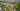The average SAT score for Yale University is 1515.

• SAT composite: 1515
• SAT math: 770

The average ACT score for Yale University is 34.

## Wesleyan University acceptance rate

Wesleyan University acceptance rate is 16.3%.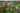The average SAT score for Wesleyan University is 1395.

• SAT composite: 1395
• SAT math: 700

The average ACT score for Wesleyan University is 32.

## Trinity College acceptance rate

Trinity College acceptance rate is 33.5%.The average SAT score for Trinity College is 1380.

• SAT composite: 1380
• SAT math: 710

The average ACT score for Trinity College is 31.

## Connecticut College acceptance rate

Connecticut College acceptance rate is 37.8%.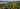The average SAT score for Connecticut College is 1358.

• SAT composite: 1358
• SAT math: 680

The average ACT score for Connecticut College is 30.

## University of Connecticut acceptance rate

University of Connecticut acceptance rate is 48.8%.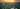The average SAT score for University of Connecticut is 1315.

• SAT composite: 1315
• SAT math: 670

The average ACT score for University of Connecticut is 29.

## Albertus Magnus College acceptance rate

Albertus Magnus College acceptance rate is 58.1%.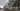The average SAT score for Albertus Magnus College is 940.

• SAT composite: 940
• SAT math: 460

The average ACT score for Albertus Magnus College is 24.

## Fairfield University acceptance rate

Fairfield University acceptance rate is 60.3%.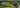The average SAT score for Fairfield University is 1270.

• SAT composite: 1270
• SAT math: 640

The average ACT score for Fairfield University is 28.

## Sacred Heart University acceptance rate

Sacred Heart University acceptance rate is 60.5%.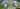The average SAT score for Sacred Heart University is 1175.

• SAT composite: 1175
• SAT math: 590

The average ACT score for Sacred Heart University is 25.

## Southern Connecticut State University acceptance rate

Southern Connecticut State University acceptance rate is 63.5%.The average SAT score for Southern Connecticut State University is 1030.

• SAT composite: 1030
• SAT math: 500

The average ACT score for Southern Connecticut State University is 20.

## Eastern Connecticut State University acceptance rate

Eastern Connecticut State University acceptance rate is 63.6%.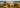The average SAT score for Eastern Connecticut State University is 1140.

• SAT composite: 1140
• SAT math: 560